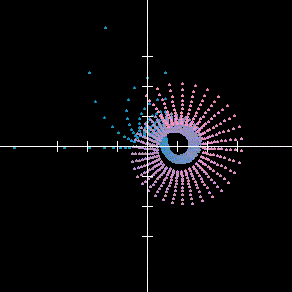## Riemann zeta function: resources

"The zeta function is probably the most challenging and mysterious object of modern mathematics, in spite of its utter simplicity."

M.C. Gutzwiller, Chaos in Classical and Quantum Mechanics (Springer-Verlag 1990)

"We may – paraphrasing the famous sentence of George Orwell – say that 'all mathematics is beautiful, yet some is more beautiful than the other'. But the most beautiful in all mathematics is the zeta function. There is no doubt about it."

Polish cosmologist Krzysztof Maslanka

"...a variety of evidence suggests that underlying Riemann's zeta function is some unknown classical, mechanical system whose trajectories are chaotic and without [time-reversal] symmetry, with the property that, when quantised, its allowed energies are the Riemann zeros. These connections between the seemingly disparate worlds of quantum mechanics and number theory are tantalising."

M. Berry, "Quantum Physics on the Edge of Chaos" (New Scientist, 19 November 1987)

Chris Caldwell: basic introduction to the Riemann zeta function

Wikipedia: Riemann zeta function

WolframMathworld: Riemann zeta function

A. Weil, "Prehistory of the zeta-function", from Number Theory, Trace Formulas and Discrete Groups, K.E. Aubert, E. Bombieri and D. Goldfeld, eds. (Academic, 1989) 1–10

R.G. Ayoub, "Euler and the zeta function", American Mathematical Monthly 81 (1974) 1067–1086

K. Devlin, "How Euler discovered the zeta function" (elementary historical introduction to the function which Riemann later extended to the complex plane)

Z. Rudnick, "Number theoretic background" (covers all the number theory necessary for a basic understanding of the Riemann Zeta Function, which is covered in its final section)

E.C. Titchmarsh, "The zeros of the Riemann zeta-function", Proc. Royal Soc. London 151 (1935) 234–255

Riemann's original eight-page paper introducing his zeta function
(English translation PDF)
[other formats]

Critical Strip Explorer v0.67, a wonderful applet produced by Raymond Manzoni for this site – explore the behaviour of the Riemann zeta function in and around the critical strip in a highly visual, interactive way. The resulting images are quite astonishing!

more applets for exploring the behaviour of the Riemann zeta function can be found on Glen Pugh's homepage, as well as here.

Noam D. Elkies' Analytic Number Theory lecture notes (Harvard University, Spring 1998)

J. Borwein, D. Bradley and R. Crandall, "Computational strategies for the Riemann zeta function", J. Comp. App. Math. 121 (2000) 247–296

J. Arias-de-Reyna, "X-Ray of Riemann zeta-function" (preprint, revised 09/2003)[an image of points in the rectangle [0,1] × [0,20] after having being transformed by the zeta function –from J. Perry's Riemann Hypothesis notes]

H. Edwards, Riemann's Zeta Function (Academic Press, 1974)

A. Ivic, The Riemann Zeta-Function: The Theory of the Riemann Zeta-Function with Applications (Wiley, 1985)

S. Patterson, An Introduction to the Theory of the Riemann Zeta-Function, (Cambridge University Press, 1988)

A.A. Karatsuba, S.M. Voronin, N. Koblitz, The Riemann Zeta-function (de Gruyter, 1992)

E. Titchmarsh, The Theory of the Riemann Zeta-Function, 2nd edition – revised by D. Heath-Brown (Oxford University Press, 1986).

a poem

number theory and physics archive      prime numbers: FAQ and tutorial
mystery      new      search      home